Published: 15 February 2017

# Research on vibration suppression of wind turbine blade based on bamboo wall three-layer damping structure

Jie Meng1
Dagang Sun2
1, 2Taiyuan University of Science and Technology, Taiyuan, China
Corresponding Authors:
Jie Meng, Dagang Sun
Views 221

#### Abstract

As wind turbine blades tend to be long and narrow, the demand for its properties of bending resistance and vibration suppression is improving day by day. Mimicking the three-layer structure (the outer skin, the middle, the inner skin) of bamboo, this paper puts forward a new damping structure with the blade as a basic layer, one transitional layer and one free damping layer added to it. The formula of the structural loss factor is deduced by vibration differential equations. Then combined with the formula, the models of airfoil flutter and damping vibration suppression and related data, the numerical simulation is done using Matlab/Simulink Software. Consequently, the comparison diagrams of swing and wave velocity responses and their displacement responses between conditions with and without the new damping structure are gained, which indicates that the property of vibration suppression of wind turbine blades with the new damping structure is significantly improved.

## 1. Introduction

Bamboo possesses unique functional gradient structures to resist wind attacks. Domestic and foreign scholars have made so many researches on bamboo structures. Ma Jianfeng , etc. compare the anti-bending properties of the bionic bamboo structure with the traditional cylindrical shell by finite element simulation analysis. They draw a conclusion that the carrying capacity of the bionic bamboo structure is 1.25 times that of the traditional structure; T. Tan , etc. put forward a series of multi-scale experiments and numerical models to analyze the functional gradient structures of bamboo, and apply Yang modulus variation finite element model to analyze the mechanical property of bamboo in a fracture toughness experiment. Meisam K. Habibi , etc. study the asymmetric bending response of bamboo under different loading conditions by multiscale mechanical characteristics with a scanning electron microscope. The results show that bamboo fibers along the wall thickness of gradient distribution mainly affect the bamboo mechanical property. So, based on the different mechanical properties of bamboo structural parts, this paper tries to apply the bamboo three-layer structure to wind turbine blades to verify whether the vibration performance of the blades with the bionic three-layer bamboo structure is significantly improved with the help of vibration differential equations, the models of airfoil flutter and damping flutter suppression and the numerical simulation.

## 2. Materials and methods

### 2.1. Bionic damping structure analysis

Bamboo internal structure is shown in Fig. 1 . Bamboo wall is composed of the outer skin, the middle and the inner skin. Their mechanical properties are closely related to their fiber volume fraction. The fiber volume fraction of the outer skin is the largest, the middle is less and the inner skin is least. It is true of their longitudinal elastic modulus and tensile strength. The reasons why bamboo has a superior ability to resist wind attacks are as follows: The outer skin has high elastic modulus and tensile strength, which can bear wind load in nature as a basic layer; Playing an important role in connecting the outer skin and the inner skin, the middle can dissipate part of the energy from wind load as a transitional layer; The inner skin can also dissipate part of the energy as a free damping layer. So, bamboo three-layer structure can be mimicked and applied to the wind turbine blade.

Fig. 1Bamboo internal structure. Note: 1 – outer skin, 2– middle, 3 – inner skin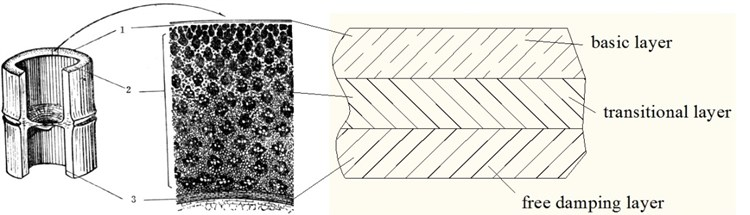### 2.2. Model analysis of the new damping structure

Mimicking the three-layer structure of bamboo, with the blade as a basic layer, one transitional layer and one free damping layer are added to form the new damping structure to suppress the blade vibration. The new damping structure is shown in Fig. 2.

Fig. 2New damping structure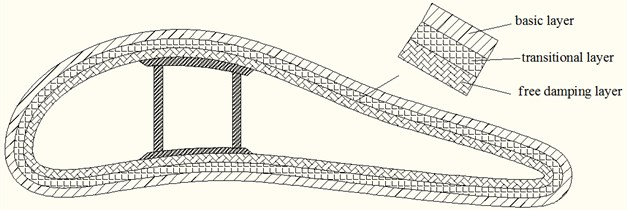Bamboo wall thickness is far less than its length, and blade shell thickness is also far less than its wingspan length. So, the blade structure can be abstracted as a beam and its bending vibration theory can be adopted to analyze the blade dynamics performance. Fig. 3 is the bending vibration parameter specification for the new damping beam .

Fig. 3Bending vibration parameter specification for the new damping beam. Note: v is transverse vibration velocity; θ is bending angle displacement; P is transverse force; M is bending moment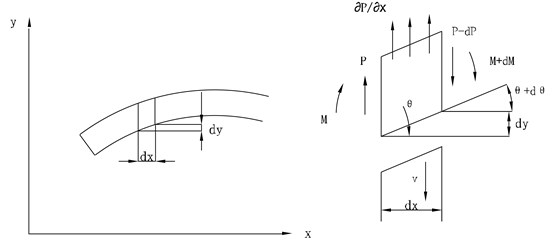In order to determine the composite bending stiffness $\stackrel{-}{B}$ of the new damping beam, the center line of the barycenter should be determined first (as is shown in Fig. 4).

When the damping structural beam is bending, the elongation $\epsilon$ of the basic, transitional and free damping layers along the longitudinal direction varies with $y$ in a linear fashion:

1
$\epsilon =\frac{yd\theta }{dx}=\frac{y}{i\omega }\frac{\partial \omega }{\partial x}.$

The normal tensile and compressive stress in $X$ direction is as follows:

2
${\sigma }_{n}={E}_{n}{\epsilon }_{n},$

where $n=$1, 2, 3 represent the basic layer, the transitional layer and the free damping layer respectively. The following formula can be gained by plugging Eq. (1) into (2):

3
${\sigma }_{n}=\frac{{E}_{n}}{i\omega }y\frac{\partial \omega }{\partial x}.$

Under pure bending, the force acting on the beam cross section along $X$ direction is equal to zero, that is:

4
${\int }_{-\left({H}_{1}-\zeta \right)}^{{H}_{2}+{H}_{3}+\zeta }\sigma dy=0.$

Fig. 4Cross-section of the new damping structure: H1, H2, H3 are the thickness of the basic layer, transitional layer and the free damping layer, and ζ is the distance between the center line of the barycenter of the damping beam and the basic layer surface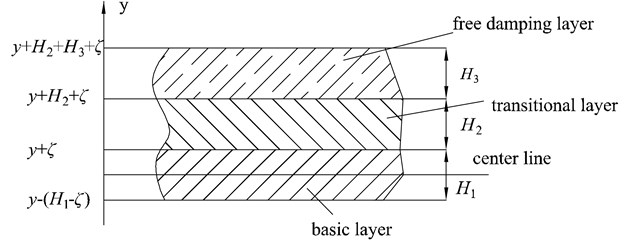The curvature $\frac{1}{i\omega }\cdot \frac{\partial \omega }{\partial x}$ is a constant. If the above formula is equal to zero, then:

5
${\int }_{-\left({H}_{1}-\zeta \right)}^{{H}_{2}+{H}_{3}+\zeta }{E}_{n}ydy=0.$

By Eq. (2), $\zeta$ can be expressed as:

6
$\zeta =\frac{1}{2}\frac{{E}_{1}{H}_{1}^{2}-{E}_{2}{H}_{2}^{2}-{E}_{3}{H}_{3}^{2}-{E}_{3}{H}_{2}{H}_{3}}{{E}_{1}{H}_{1}+{E}_{2}{H}_{2}+{E}_{3}{H}_{3}}.$

After $\zeta$ is determined, bending moment $M$ can be expressed as:

7
$M={\int }_{-\left({H}_{1}-\zeta \right)}^{{H}_{2}+{H}_{3}+\zeta }y\sigma dy.$

Plugging formula $M=\frac{\stackrel{-}{B}}{i\omega }\cdot \frac{\partial \omega }{\partial x}$ and Eq. (3) into (7), the following can be gained:

8
$\stackrel{-}{B}={\int }_{-\left({H}_{1}-\zeta \right)}^{{H}_{2}+{H}_{3}+\zeta }{E}_{n}{y}^{2}dy$
$=\frac{{E}_{1}{H}_{1}^{3}}{3}\left[{\stackrel{-}{e}}_{2}\left(3{h}_{1}^{2}{h}_{2}+{h}_{2}^{3}+3{h}_{2}\frac{{\zeta }^{2}}{{H}_{1}^{2}}+3{h}_{1}{h}_{2}^{2}+6{h}_{1}{h}_{2}\frac{\zeta }{{H}_{1}}+3{h}_{2}^{2}\frac{\zeta }{{H}_{1}}\right)\right$
$+{\stackrel{-}{e}}_{1}\left({h}_{1}^{3}+3{h}_{1}^{2}\frac{\zeta }{{H}_{1}}+3{h}_{1}\frac{{\zeta }^{2}}{{H}_{1}^{2}}\right)+1-3\frac{\zeta }{{H}_{1}}+3\frac{{\zeta }^{2}}{{H}_{1}^{2}}],$

where ${\stackrel{-}{e}}_{1}={\stackrel{-}{E}}_{2}/{E}_{1}$, ${\stackrel{-}{e}}_{2}={\stackrel{-}{E}}_{3}/{E}_{1}$, ${h}_{1}={H}_{2}/{H}_{1}$, ${h}_{2}={H}_{3}/{H}_{1}$.

Eq. (6) can also be expressed as:

9
$\zeta =\frac{{H}_{1}-{\stackrel{-}{e}}_{1}{h}_{1}{H}_{2}-{\stackrel{-}{e}}_{2}{h}_{2}\left({H}_{3}+{H}_{2}\right)}{2\left(1+{\stackrel{-}{e}}_{1}{h}_{1}+{\stackrel{-}{e}}_{2}{h}_{2}\right)}.$

Plugging Eq. (9) into (8), the following can be gained:

10
$\stackrel{-}{B}=\frac{{B}_{1}}{1+{\stackrel{-}{e}}_{1}{h}_{1}+{\stackrel{-}{e}}_{2}{h}_{2}}\left[1+2{\stackrel{-}{e}}_{1}\left(2{h}_{1}+3{h}_{1}^{2}+2{h}_{1}^{3}\right)+2{\stackrel{-}{e}}_{2}\left(2{h}_{2}+6{h}_{1}^{2}{h}_{2}+2{h}_{2}^{3}+6{h}_{1}{h}_{2}^{2}\right$
$+6{h}_{1}{h}_{2}+3{h}_{2}^{2}\right)+4{\stackrel{-}{e}}_{1}^{2}{h}_{1}^{4}+12{\stackrel{-}{e}}_{2}^{2}\left({h}_{1}^{2}{h}_{2}^{2}+{h}_{1}{h}_{2}^{3}\right)+4{\stackrel{-}{e}}_{1}{\stackrel{-}{e}}_{2}\left(4{h}_{1}^{3}{h}_{2}+{h}_{1}{h}_{2}^{3}+{h}_{2}^{4}+3{h}_{1}^{2}{h}_{2}^{2}\right)],$

where $\stackrel{-}{B}$ is the composite bending stiffness of the damping structural beam. ${B}_{1}$ is the bending stiffness of the ordinary beam.

Compared with the loss factor ${\beta }_{2}$ and ${\beta }_{3}$ of the viscoelastic damping materials on the transitional layer and free damping layer, the loss factor ${\beta }_{1}$ of the basic layer is so small that it can be ignored. Also, $\stackrel{-}{B}=B\left(1+i\eta \right)$, ${\stackrel{-}{e}}_{1}=e\left(1+i{\beta }_{2}\right)$, ${\stackrel{-}{e}}_{2}=e\left(1+i{\beta }_{3}\right)$, and $\eta$ is the loss factor of the new damping structure. So, the following can be gained:

11
$\frac{B}{{B}_{1}}\left(1+i\eta \right)=\frac{1}{1+{e}_{1}\left(1+i{\beta }_{2}\right){h}_{1}+{e}_{2}\left(1+i{\beta }_{3}\right){h}_{2}}\left[1+2{e}_{1}\left(1+i{\beta }_{2}\right)a\right+2{e}_{2}\left(1+i{\beta }_{3}\right)b$
$+4{e}_{1}^{2}{\left(1+i{\beta }_{2}\right)}^{2}{h}_{1}^{4}+12{e}_{2}^{2}{\left(1+i{\beta }_{3}\right)}^{2}c+4{e}_{1}\left(1+i{\beta }_{2}\right){e}_{2}\left(1+i{\beta }_{3}\right)d],$

where $a$, $b$, $c$ and $d$:

$a=2{h}_{1}+3{h}_{1}^{2}+2{h}_{1}^{3},b=2{h}_{2}+6{h}_{1}^{2}{h}_{2}+2{h}_{2}^{3}+6{h}_{1}{h}_{2}^{2}+6{h}_{1}{h}_{2}+3{h}_{2}^{2},$
$c={h}_{1}^{2}{h}_{2}^{2}+{h}_{1}{h}_{2}^{3},d=4{h}_{1}^{3}{h}_{2}+{h}_{1}{h}_{2}^{3}+{h}_{2}^{4}+3{h}_{1}^{2}{h}_{2}^{2}.$

Omitting ${\beta }_{2}{\beta }_{3}$, ${\beta }_{2}^{2}$ and ${\beta }_{2}^{2}$, the real component can be expressed as:

12
$\frac{B}{{B}_{1}}=\frac{1}{{\left(1+{e}_{1}{h}_{1}+{e}_{2}{h}_{2}\right)}^{2}}\left[1+{e}_{1}\left(2a+1\right)+{e}_{2}\left(2b+1\right)+2{e}_{1}^{2}\left(2{h}_{1}^{4}+a+2{e}_{1}{h}_{1}^{4}\right)\right$
$+2{e}_{2}^{2}\left(6c+b+6{e}_{2}c+2{e}_{1}{e}_{2}\left(2d+a+b+2{e}_{2}d+6{e}_{2}c\right)+4{e}_{1}^{2}{e}_{2}\left(1+{\beta }_{2}d+{h}_{1}^{4}\right)].$

Omitting ${\beta }_{2}{\beta }_{3}$, ${\beta }_{2}^{2}$ and ${\beta }_{2}^{2}$, the imaginary component can be expressed as:

13
$i\frac{B}{{B}_{1}}\eta =\frac{i}{{\left(1+{e}_{1}{h}_{1}+{e}_{2}{h}_{2}\right)}^{2}}\left\{{e}_{1}{\beta }_{2}\left(2a-1\right)+{e}_{2}{\beta }_{3}\left(2b-1\right)+36{e}_{2}^{2}{\beta }_{3}c+12{e}_{1}^{2}{h}_{1}^{4}{\beta }_{2}\right\$
$\begin{array}{ll}& +2{e}_{1}{e}_{2}\left[2\left({\beta }_{2}+{\beta }_{3}\right)d+\left(a-b\right)\left({\beta }_{2}-{\beta }_{3}\right)\right]+4\end{array}{e}_{1}^{2}{e}_{2}\left[{\beta }_{3}d+{h}_{1}^{4}\left(2{\beta }_{2}-{\beta }_{3}\right)\right]$
$\begin{array}{ll}& +4{e}_{1}{e}_{2}^{2}\left(6{\beta }_{3}c+{\beta }_{2}d-3{\beta }_{2}c\right)}\end{array}.$

Plugging Eq. (12) into (13) and omitting the higher-order item, the formula for $\eta$ can be expressed as:

14
$\eta =\frac{{e}_{1}{\beta }_{2}\left(2a-1\right)+{e}_{2}{\beta }_{3}\left(2b-1\right)}{1+{e}_{1}\left(2a+1\right)+{e}_{2}\left(2b+1\right)}.$

From Eqs. (12) and (14), if the material elastic modulus of the new damping structure (${e}_{1}={E}_{2}/{E}_{1}$ and ${e}_{2}={E}_{3}/{E}_{1}$), the thickness ratios (${h}_{1}={H}_{2}/{H}_{1}$and ${h}_{2}={H}_{3}/{H}_{1}$), the loss factors (${\beta }_{2}$ and ${\beta }_{3}$), the bending stiffness of the basic layer without the damping structure (${B}_{1}$) are known, the composite bending stiffness of the new damping structure ($B$) and the loss factor $\eta$ can be calculated.

### 2.3. Models of airfoil flutter and damping vibration suppression

This study sets up the two degrees of freedom airfoil vibration dynamic model based on typical sections. Fig. 5 is the two degrees of freedom airfoil model and its coordinates.

Fig. 5Airfoil elastic supporting structure and coordinate system. Note: x stands for the vertical axis and the displacement of blade section. y stands for the horizontal axis. θ1 stands for the torsional angle of blade section. L stands the lift force. D stands for the drag force. The blade is supported by a twist spring kθ and a pull-press spring kx at the center of torsion E. The center of mass is G. The center of torsion is e. The aerodynamic center is A. A and G are δ apart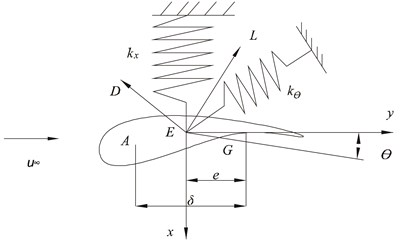The classic vibration of blades is a sort of strong aeroelastic unstable phenomenon. Its main element is the blade torsion-bending-coupled vibration . The two degrees of freedom airfoil dynamic Eq. (15) is deduced by Lagrange equation:

15
$\left[\begin{array}{ll}{J}_{G}& 0\\ 0& m\end{array}\right]\left\{\begin{array}{l}{\stackrel{¨}{\theta }}_{1}\\ \stackrel{¨}{x}\end{array}\right\}+\left[\begin{array}{cc}{k}_{\theta }+{e}^{2}{k}_{x}& -e{k}_{x}\\ -e{k}_{x}& {k}_{x}\end{array}\right]\left\{\begin{array}{c}{\theta }_{1}\\ x\end{array}\right\}=\left\{\begin{array}{c}{M}_{1}\\ F\end{array}\right\},$

where ${J}_{G}$ is the airfoil moment of inertia about $G$, ${M}_{1}$ is the torque acted on $G$, and $F$ is the aerodynamic force acted on $G$. $e$ is the distance between $G$ and $E$. $m$ is the mass of airfoil cross section. ${k}_{x}=3{E}_{1}{I}_{\eta }/{r}^{3}$ and ${k}_{\theta }={E}_{2}{I}_{p}/r$ are calculated by blade material stiffness and airfoil parameters . (${E}_{1}$ and ${E}_{2}$ are blade material shear modulus and elastic modulus. ${I}_{P}$ is the airfoil polar moment of inertia relative to $G$. $I\eta$ is the airfoil polar moment of inertia relative to the centroidal axis. $r$ is the distance between the airfoil cross section and the axis of rotation).

From the aerodynamic theory:

16
$\left\{\begin{array}{l}{M}_{1}=0.5\rho {w}^{2}c\delta \left({C}_{l}\mathrm{c}\mathrm{o}\mathrm{s}\alpha +{C}_{d}\mathrm{s}\mathrm{i}\mathrm{n}\alpha \right),\\ F=0.5\rho {w}^{2}c\left({C}_{l}\mathrm{c}\mathrm{o}\mathrm{s}\alpha +{C}_{d}\mathrm{s}\mathrm{i}\mathrm{n}\alpha \right),\end{array}\right\$

where $c$ is the airfoil chord length, $w$ is the blade cross section relative velocity, and $\rho$ is the air density. Eq. (16) can be also expressed as:

17
$\left\{\begin{array}{c}{M}_{1}\\ F\end{array}\right\}=\left[\begin{array}{ll}{s}_{11}& {s}_{12}\\ {s}_{21}& {s}_{22}\end{array}\right]\left\{\begin{array}{l}{\theta }_{1}\\ x\end{array}\right\}-\left[\begin{array}{ll}{d}_{11}& {d}_{12}\\ {d}_{21}& {d}_{22}\end{array}\right]\left\{\begin{array}{l}{\stackrel{˙}{\theta }}_{1}\\ \stackrel{˙}{x}\end{array}\right\},$

where ${K}_{A}=\left[\begin{array}{ll}{s}_{11}& {s}_{12}\\ {s}_{21}& {s}_{22}\end{array}\right]$, ${C}_{A}=\left[\begin{array}{ll}{d}_{11}& {d}_{12}\\ {d}_{21}& {d}_{22}\end{array}\right]$ are the aerodynamic stiffness and damping of aerodynamic center.

Blades don’t vibrate under steady flow. Suppose blade cross section relative velocity is ${w}_{0}$, attack angle is ${\alpha }_{0}$, and setting angle is $\gamma$ at the steady-state moment. Take the airfoil at the distance of r away from the blade root as the research object. The following formula is derived from the airfoil velocity at the steady-state moment:

18
${w}_{0}=\sqrt{{v}_{0}^{2}+{\left(\mathrm{\Omega }r\right)}^{2}{\left(1+a\text{'}\right)}^{2}}.$

Among them, ${v}_{0}$ is velocity in wind wheel plane, ${v}_{0}={u}_{\infty }\left(1+a\right)$, and ${u}_{\infty }$ is wind velocity, $a$ is axial inducible factor, $\mathrm{\Omega }$ is blade wheel rotational velocity, $a\text{'}$ is tangential inducible factor

When the wind velocity changes, blades vibrate, the tangential velocity increment is $-\stackrel{˙}{x}\mathrm{s}\mathrm{i}\mathrm{n}\gamma$, and the axial velocity increment is $\stackrel{˙}{x}\mathrm{c}\mathrm{o}\mathrm{s}\gamma$, and the torsional angle of blade section is –${\theta }_{1}$, the blade cross section relative velocity is as follows:

19
$w=\sqrt{{\left({v}_{0}+\stackrel{˙}{x}\mathrm{c}\mathrm{o}\mathrm{s}\mathrm{\gamma }\right)}^{2}+{\left(\mathrm{\Omega }r-\stackrel{˙}{x}\mathrm{s}\mathrm{i}\mathrm{n}\mathrm{\gamma }\right)}^{2}{\left(1+a\text{'}\right)}^{2}}.$

Then, the attack angle $\alpha ={\alpha }_{0}-{\theta }_{1}$, the inflow angle $\phi =\alpha +\gamma$, and its tangent function is as follows:

20
$\mathrm{t}\mathrm{a}\mathrm{n}\phi =\frac{{v}_{0}+\stackrel{˙}{x}\mathrm{c}\mathrm{o}\mathrm{s}\gamma }{\left(\mathrm{\Omega }r-\stackrel{˙}{x}\mathrm{s}\mathrm{i}\mathrm{n}\gamma \right)\left(1+a\text{'}\right)}.$

As both $x$ and ${\stackrel{˙}{\theta }}_{1}$ have no relation with aerodynamic , so ${K}_{A}=\left[\begin{array}{ll}{s}_{11}& 0\\ {s}_{21}& 0\end{array}\right]$, ${C}_{A}=\left[\begin{array}{ll}0& {d}_{12}\\ 0& {d}_{22}\end{array}\right]$.

Plugging ${K}_{A}$ and ${C}_{A}$, the airfoil flutter equation is expressed as:

21
$P\stackrel{¨}{X}+C\stackrel{˙}{X}+KX=0,$

where $P=\left[\begin{array}{ll}{J}_{G}& 0\\ 0& m\end{array}\right]$, $C={C}_{A}+{C}_{S}$, $K={K}_{A}+{K}_{S}$. ${K}_{s}$ and ${C}_{s}$ are the structural stiffness and structural damping:

${K}_{S}=\left[\begin{array}{cc}{k}_{\theta }+{e}^{2}{k}_{x}& -e{k}_{x}\\ -e{k}_{x}& {k}_{x}\end{array}\right],{C}_{S}=\left[\begin{array}{ll}{c}_{s11}& {c}_{s12}\\ {c}_{s21}& {c}_{s22}\end{array}\right],{K}_{A}=\left[\begin{array}{ll}{s}_{11}& 0\\ {s}_{21}& 0\end{array}\right],{C}_{A}=\left[\begin{array}{ll}0& {d}_{12}\\ 0& {d}_{22}\end{array}\right].$

where:

${s}_{11}=\frac{1}{2}\delta \rho {w}_{0}^{2}c\left[-{C}_{l}^{\text{'}}\left({\alpha }_{0}\right)\mathrm{c}\mathrm{o}\mathrm{s}\alpha +{C}_{l}\left({\alpha }_{0}\right)\mathrm{s}\mathrm{i}\mathrm{n}{\alpha }_{0}-{C}_{d}^{\text{'}}\left({\alpha }_{0}\right)\mathrm{s}\mathrm{i}\mathrm{n}\alpha +{C}_{d}\left({\alpha }_{0}\right)\mathrm{c}\mathrm{o}\mathrm{s}{\alpha }_{0}\right],$
${s}_{11}=\frac{1}{2}\delta \rho {w}_{0}^{2}c\left[-{C}_{l}^{\text{'}}\left({\alpha }_{0}\right)\mathrm{c}\mathrm{o}\mathrm{s}\alpha +{C}_{l}\left({\alpha }_{0}\right)\mathrm{s}\mathrm{i}\mathrm{n}{\alpha }_{0}-{C}_{d}^{\text{'}}\left({\alpha }_{0}\right)\mathrm{c}\mathrm{o}\mathrm{s}\alpha +{C}_{d}\left({\alpha }_{0}\right)\mathrm{s}\mathrm{i}\mathrm{n}{\alpha }_{0}\right],$
${d}_{12}=\rho c\delta \left({C}_{l}\mathrm{c}\mathrm{o}\mathrm{s}{\alpha }_{0}+{C}_{d}\mathrm{s}\mathrm{i}\mathrm{n}{\alpha }_{0}\right)\left[{v}_{0}\mathrm{c}\mathrm{o}\mathrm{s}\beta -\mathrm{\Omega }r{\left(1+a\text{'}\right)}^{2}\mathrm{s}\mathrm{i}\mathrm{n}\gamma \right]$
$+\frac{1}{2}\rho \delta c\left(1+a\text{'}\right)\left(\Omega rcos\gamma +{v}_{0}\mathrm{s}\mathrm{i}\mathrm{n}\gamma \right)\left[\frac{d{C}_{l}\left(\phi -\gamma \right)}{d\phi }\right\mathrm{c}\mathrm{o}\mathrm{s}\left(\delta -\gamma \right)-{C}_{l}\left(\phi -\gamma \right)\mathrm{s}\mathrm{i}\mathrm{n}\left(\phi -\gamma \right)$
$+\frac{d{C}_{d}\left(\phi -\gamma \right)}{d\phi }\mathrm{s}\mathrm{i}\mathrm{n}\left(\phi -\gamma \right)+{C}_{d}\left(\phi -\gamma \right)\mathrm{c}\mathrm{o}\mathrm{s}\left(\phi -\gamma \right)],$
${d}_{22}=\rho c\delta \left({C}_{l}\mathrm{c}\mathrm{o}\mathrm{s}{\alpha }_{0}+{C}_{d}\mathrm{s}\mathrm{i}\mathrm{n}{\alpha }_{0}\right)\left[{v}_{0}\mathrm{c}\mathrm{o}\mathrm{s}\gamma -\mathrm{\Omega }r{\left(1+a\text{'}\right)}^{2}\mathrm{s}\mathrm{i}\mathrm{n}\gamma \right]$
$+\frac{1}{2}\rho c\left(1+a\text{'}\right)\left(\mathrm{\Omega }r\mathrm{c}\mathrm{o}\mathrm{s}\gamma +{v}_{0}\mathrm{s}\mathrm{i}\mathrm{n}\gamma \right)\left[\frac{d{C}_{l}\left(\phi -\gamma \right)}{d\phi }\right\mathrm{c}\mathrm{o}\mathrm{s}\left(\delta -\gamma \right)-{C}_{l}\left(\phi -\gamma \right)\mathrm{s}\mathrm{i}\mathrm{n}\left(\phi -\gamma \right)$
$+\frac{d{C}_{d}\left(\phi -\gamma \right)}{d\phi }\mathrm{s}\mathrm{i}\mathrm{n}\left(\phi -\gamma \right)+{C}_{d}\left(\phi -\gamma \right)\mathrm{c}\mathrm{o}\mathrm{s}\left(\phi -\gamma \right)].$

If the material is assumed to be isotropic, the equivalent complex elastic stiffness of the structure can be expressed as:

22
${\stackrel{-}{E}}_{eq}={E}_{eq}^{\text{'}}+i{E}_{eq}^{\text{'}\text{'}}.$

As $\stackrel{-}{B}={\stackrel{-}{E}}_{eq}{I}_{z}$, the real and imaginary parts at both sides of the equation are equal. So, the following formulas can be deduced as:

23
$B={E}_{eq}^{\text{'}}{I}_{z},$
24
$B\eta ={E}_{eq}^{\text{'}\text{'}}{I}_{z}.$

Among them, ${I}_{z}$ is the moment of inertia of the damping structure to the neutral axis. The equivalent storage modulus ${E}_{eq}^{\text{'}}$ and loss modulus ${E}_{eq}^{\text{'}\text{'}}$ can be calculated by Eqs. (23) and (24). So, with the damping material, the damping matrix in Eq. (21) can be expressed as:

25
${C}_{s}=\left[\begin{array}{cc}\frac{\xi B\eta }{{I}_{z}}& 0\\ 0& \frac{B\eta }{{I}_{z}}\end{array}\right].$

In the matrix, $\xi =$0.5 ($1+\lambda$), where $\lambda$ is Poisson ratio. The analysis of the vibration suppression property of blades with the new damping structure can be done by plugging Eqs. (25) in (21).

## 3. Results and discussion

### 3.1. Numerical simulation experiment

A 600 kw wind turbine is selected in this simulation experiment, the blade shape of which adopts FX-77-153 aeronautics airfoil and the material is fiberglass. The main design parameters are shown in Table 1. The airfoil section main design parameters are shown in Table 2 . The lift and drag coefficient functions are obtained through polynomial fitting within the scope of the designed attack angle:

26
${C}_{l}\left(\alpha \right)=0.1043\alpha -0.0116,$
27
${C}_{d}\left(\alpha \right)=0.57×1{0}^{5}{\alpha }^{3}+0.13×1{0}^{3}{\alpha }^{2}-0.23×1{0}^{3}\alpha +0.0085,$

where ${C}_{l}$ is the lift coefficient, ${C}_{d}$ is the drag coefficient. The designed attack angle $\alpha =$ 10°, so ${C}_{l}\left(\alpha \right)=$ 1.0314, ${C}_{d}\left(\alpha \right)=$ 0.0248, ${C}_{l}^{\text{'}}\left(\alpha \right)=$ 0.1043, ${C}_{d}^{\text{'}}\left(\alpha \right)=$ 0.0028. The blade section parameters at the radius of 20 % to 30 % from the wing root was selected as the two degrees of freedom airfoil parameters, so $r=$5 m, $a=$ 0.38, $a\text{'}=$ 0.1128  based on empirical models.

The matrixes of Eqs. (21) are calculated by the parameters in Table 1 and 2 and the related formulas:

$P=\left[\begin{array}{cc}10.40& 0\\ 0& 185.83\end{array}\right],{K}_{A}=\left[\begin{array}{cc}-22& 0\\ -130& 0\end{array}\right],{C}_{A}=\left[\begin{array}{ll}0& 1.4\\ 0& 8.1\end{array}\right],$
$Ks=\left[\begin{array}{cc}5.53×1{0}^{6}& -0.617×1{0}^{3}\\ -0.617×1{0}^{3}& 0.0115×1{0}^{6}\end{array}\right]$

Material parameters for the transitional layer is as follows: ${\beta }_{2}=$0.5, ${E}_{2}=$2.9×108 Pa, $\lambda =$0.32; Material parameters for the free damping layer is as follows: ${\beta }_{3}=$1.2, ${E}_{3}=$1.14×108 Pa, $\lambda =$0.5 .Without considering the temperature efficiency effect of damping materials, the structural damping $Cs=\left[\begin{array}{cc}5145000& 0\\ 0& 1710000\end{array}\right]$. The properties of blade vibration and damping suppression are numerically simulated based on Eq. (21) by building simulation models in software Matlab/Simulink. Based on the ONERA non-linear aerodynamic model, the numerical simulations for the ordinary blade and the blade with the new damping structure are done under four different velocities: start-up wind velocity ${V}_{1}=$4 m/s, rated wind velocity ${V}_{2}=$15 m/s, machine halt wind velocity ${V}_{3}=$25 m/s and dangerous wind velocity ${V}_{4}=$45 m/s. Fig. 6 and 7 show the swing and wave velocity responses and displacement responses of the two blades under four different wind velocities.

Table 1Wind turbine main design parameters

 Designed item Output power / kW Power coefficient Rotor velocity / rad·s-1 Rotor diameter / m Designed attack angle / (°) Setting angle / (°) Value 600 0.4 7 40 10 1.63

Table 2Foil section main design parameters

 Airfoil parameters Chord length / m Cross section mass per unit / kg Moment of inertia / Kg·m-2 Axial moment of inertia / cm4 Polar moment of inertia / cm4 Value 1.073 185.83 10.4 17364 673110

### 3.2. Analysis

From Fig. 6 and 7, as the wind velocity increases, the ordinary blade swing and wave amplitudes are increasing, and the wave displacement increases obviously; When the start-up velocity is rising to the machine halt velocity, the blade swing and wave amplitude are increasing slowly; When it is over the machine halt velocity, the blade vibration amplitude are increasing rapidly and significantly; When it reaches to the dangerous velocity, the blade cracks and even ruptures because the vibration amplitude exceeds the permitted value. For the damping blade, As the wind velocity increases, its swing and wave amplitude are also increasing, and the wave displacement increases within a small range; When the start-up velocity is rising to the machine halt velocity, its swing and wave amplitude are also increasing slowly; When it is over the machine halt velocity, the blade vibration amplitude increasement slows down significantly; When it reaches to the dangerous velocity, the blade doesn’t crack and rupture because the vibration amplitude is within the permitted value.

Fig. 6Ordinary and damping blades velocity responses. Note: compared with the ordinary blade, the standard deviation of the damping blade swing velocity decreases by 37.9 %, 35.0 %, 30.8 %, 25.0 % respectively; those of the wave velocity decreases by 51.1 %, 47.9 %, 43.7 % and 37.1 % respectively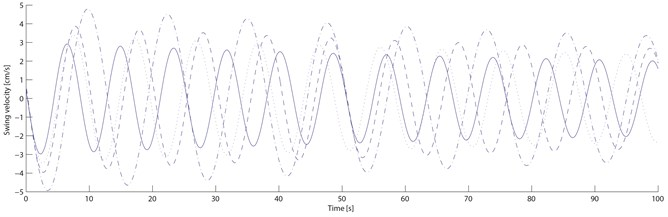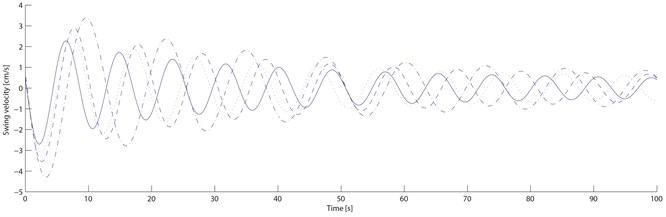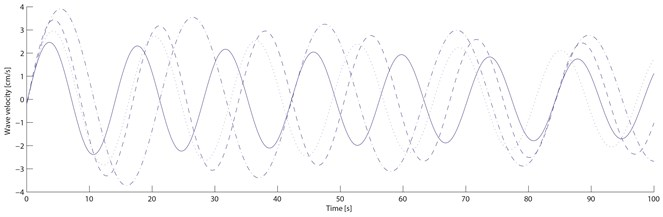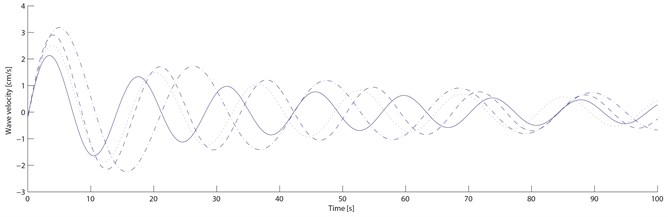Four structural loss factors (${\eta }_{1}=$0.335, ${\eta }_{2}=$0.323, ${\eta }_{3}=$0.289, ${\eta }_{4}=$0.241) of the damping blade under four different velocities are calculated by the swing and wave data. From Table 3, compared with the ordinary blade, the standard deviation of the damping blade swing velocity decreases by 37.9 %, 35.0 %, 30.8 %, 25.0 % respectively; those of the swing displacement decreases by 37.9 %, 34.8 %, 30.8 % and 25.2 % respectively; those of the wave velocity decreases by 51.1 %, 47.9 %, 43.7 % and 37.1 % respectively; those of the wave displacement decreases by 51.1 %, 48.1 %, 43.6 % and 37.1 % respectively. With the transitional and free damping layers on the blade, the damping blade vibration amplitude is smaller than the ordinary blade because of its lower natural frequency and higher structural loss factor. The damping blade vibration amplitude decreases more quickly than the ordinary within the same period because the transitional and free damping layers turn part of the vibration energy into heat energy. Through the numerical simulation experiment, it can be concluded that the vibration amplitude of the damping blade decreases sharply within a number of vibration periods, which can delay the fatigue damage process effectively, and the property of blade vibration suppression with the new damping structure can be improved significantly.

Fig. 7Ordinary and damping blades displacement responses. Note: compared with the ordinary blade, those of the swing displacement decreases by 37.9 %, 34.8 %, 30.8 % and 25.2 % respectively; those of the wave displacement decreases by 51.1 %, 48.1 %, 43.6 % and 37.1 % respectively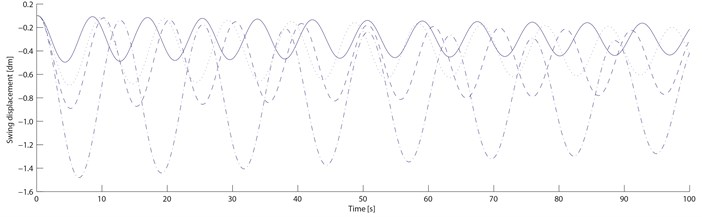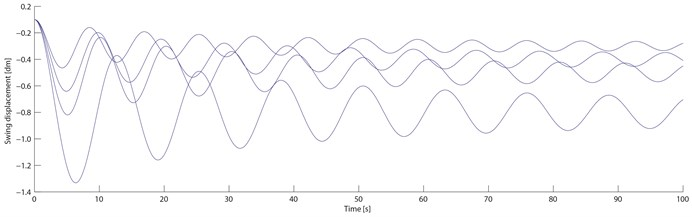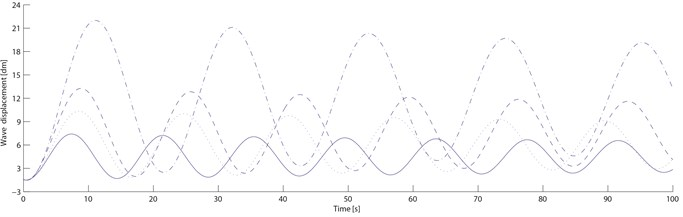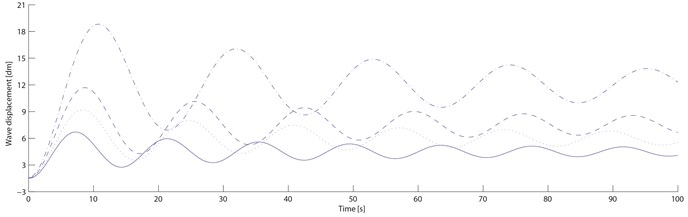Table 3Simulation results

 Operatingconditions Evaluationindex Swing Wave Ordinary Damping Ordinary Damping $D$×10-2 $V$×10-2 $D$×10-2 $V$×10-2 $D$×10-1 $V$×10-1 $D$×10-1 $V$×10-1 Set-up wind Max. 0.00 0.20 0.00 0.20 0.60 0.18 0.60 0.18 Min. –0.41 –0.21 –0.40 –0.20 0.00 –0.19 0.00 –0.17 SD. 0.09 0.09 0.07 0.07 0.17 0.103 0.14 0.08 Ratedwind Max. 0.00 0.23 0.00 0.22 0.79 0.21 0.78 0.20 Min. –0.54 –0.24 –0.53 –0.23 0.00 –0.21 0.00 –0.19 SD. 0.13 0.18 0.10 0.09 0.22 0.12 0.19 0.09 Machine haltwind Max. 0.00 0.28 0.00 0.27 1.15 0.25 1.13 0.25 Min. –0.78 –0.29 –0.77 –0.29 0.00 –0.24 0.00 –0.23 SD. 0.20 0.15 0.16 0.11 0.34 0.15 0.29 0.13 Dangerouswind Max. 0.00 0.37 0.00 0.36 2.00 0.34 1.99 0.33 Min. –1.36 –0.39 –1.34 –0.38 0.00 –0.33 0.00 –0.32 SD. 0.38 0.21 0.31 0.17 0.62 0.21 0.54 0.18 Note: $D$ stands for displacement (m); $V$ stands for velocity (m/s). SD stands for standard deviation

## 4. Conclusions

Mimicking the three-layer bamboo structure (the outer skin, the middle, the inner skin), this paper puts forward a new structure (a basic layer, a transitional layer and a free damping layer) applied on wind turbine blades. It deduces the formula for $\zeta$ that is the distance between the center line of the barycenter of the damping beam and the basic layer surface, the formula for the composite bending stiffness of the damping beam by vibration differential equations, and the formula for structural loss factor $\eta$. Based on the models of airfoil flutter and damping vibration suppression, the numerical simulation experiment on a 600 kw wind turbine is done using Matlab/Simulink Software. Consequently, the comparison diagrams of swing and wave velocity responses and their displacement responses between conditions with and without the new damping structure are gained. From the diagrams, as the wind velocity increases, both the ordinary blade and the damping blade swing and wave amplitudes are increasing, but the ordinary blade waving displacement increases obviously, and the damping blade increases within a small range; When the start-up velocity is rising to the machine halt velocity, both the blades swing and wave amplitude are increasing slowly; When it is over the machine halt velocity, the ordinary blade vibration amplitude are increasing rapidly and significantly, but the damping blade vibration amplitude increasement slows down significantly; When it reaches to the dangerous velocity, the ordinary blade cracks and even ruptures because its vibration amplitude exceeds the permitted value, but the damping blade doesn’t crack or rupture because its vibration amplitude is within the permitted value. All the above indicate that the vibration amplitude of the damping blade decreases sharply within a number of vibration periods, which can delay the fatigue damage process effectively and the property of vibration suppression of wind turbine blades with the new damping structure is significantly improved.

07 July 2016
Accepted
08 December 2016
Published
15 February 2017
SUBJECTS
Mechanical vibrations and applications
Keywords